# How to read an imperial vernier caliper?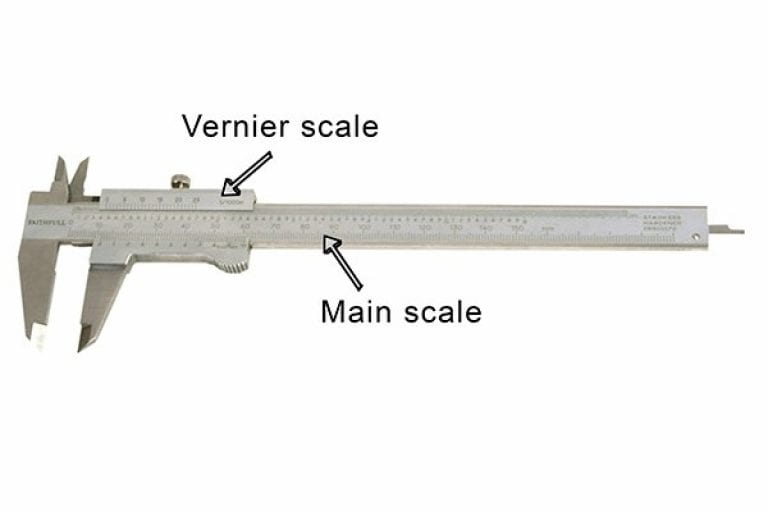Although the vernier caliper can be used to measure a variety of different dimensions, the way in which it is read is always the same.

The reading on a vernier caliper is a combination of the value shown on the main scale and the value indicated on the sliding vernier scale.

## Guide to Reading an Imperial Vernier Caliper

### Step 1 - Look at the main scale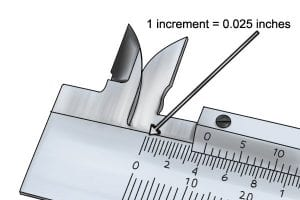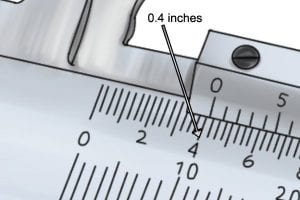When taking a measurement, you should first read the value on the main scale. On an imperial vernier caliper, this will be given in inches and tenths of an inch.

The smallest value that can read from the main scale is 0.025 inches (indicated by a single increment).

The value on the main scale is the number immediately to the left of the 0 marker of the vernier scale. In this instance, this value is 0.4 inches.

As the zero is half way between 0.4 and 0.425, we know that the distance between the jaws is somewhere between these two values.

When taking a measurement, you should first read the value on the main scale. On an imperial vernier caliper, this will be given in inches and tenths of an inch.

The smallest value that can read from the main scale is 0.025 inches (indicated by a single increment).The value on the main scale is the number immediately to the left of the 0 marker of the vernier scale.

In this instance, this value is 0.4 inches.

As the zero is half way between 0.4 and 0.425, we know that the distance between the jaws is somewhere between these two values.### Step 2 - Look at the vernier scale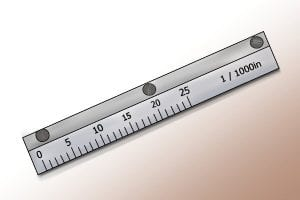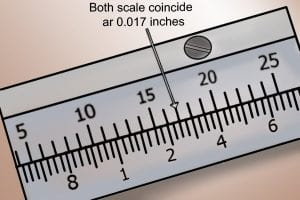Next, look at the vernier scale.

The vernier scale of an imperial caliper has a measuring range of 0.025 inches, and is graduated in 25 increments.

Each increment represents 0.001 inches (a thousandth of an inch).

When reading the vernier scale, identify the increment that lines up most accurately with an increment on the main scale. This value will make up the second part of your measurement.

In the example, the increments of both scales coincide at 17 thousandths of an inch (0.017).

Next, look at the vernier scale.

The vernier scale of an imperial caliper has a measuring range of 0.025 inches, and is graduated in 25 increments. Each increment represents 0.001 inches (a thousandth of an inch).When reading the vernier scale, identify the increment that lines up most accurately with an increment on the main scale. This value will make up the second part of your measurement.

In the example, the increments of both scales coincide at 17 thousandths of an inch (0.017).### Step 3 - Add values together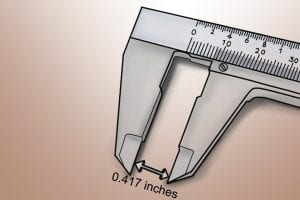To get your total reading, add both the value from the main scale and the value from the vernier scale together.

e.g. 0.4 + 0.017 = 0.417

So, the distance between the jaws of the vernier caliper is 0.417 inches.

To get your total reading, add both the value from the main scale and the value from the vernier scale together.

e.g. 0.4 + 0.017 = 0.417

So, the distance between the jaws of the vernier caliper is 0.417 inches.